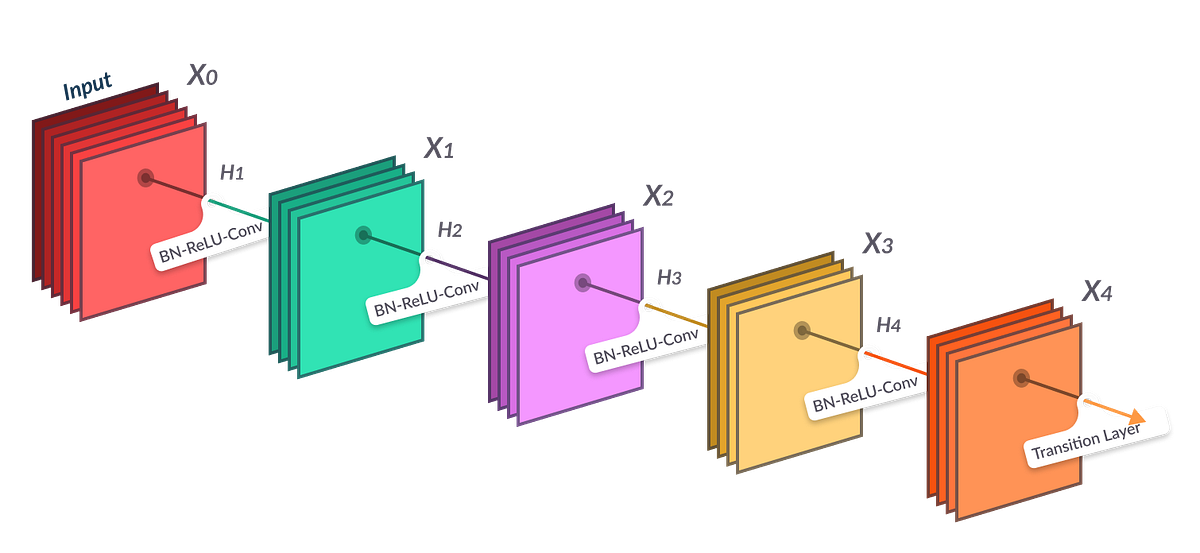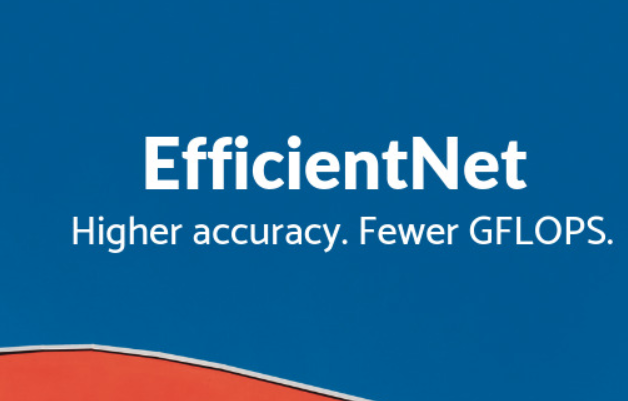# How to add additional layers in a pre-trained model using PytorchMost of us find that it is very difficult to add additional layers and generate connections between the model and additional layers . But , here I am going to make it simple . So that , everyone can get benfit out of it . Just read this out once and we will be good to go .

Most of us find that it is very difficult to add additional layers and generate connections between the model and additional layers . But , here I am going to make it simple . So that , everyone can get benfit out of it . Just read this out once and we will be good to go .

So , here I am going to use the architechture of two small models (EfficientNet_b0 & ResNet18) as our example to understand the topic .

### EfficientNet_b0 →First of all we will install the pre-trained model

``!pip install efficientnet_pytorch``

then if we look in the GitHub of efficientNet of Pytorch we will find import for this

``from efficientnet_pytorch import EfficientNet``

finally we will define our own class

``class EfficientNet_b0(nn.Module):``

After that we define the constructor for our class

``````def __init__(self):
super(EfficientNet_b0, self).__init__()

##  where this line super(EfficientNet_b0, self).__init__() is used to inherit nn.Module used above.``````

After that we will load the Pre-trained EfficientNet Model .

``self.model = efficientnet_pytorch.EfficientNet.from_pretrained('efficientnet-b0')``

and finally I dediced to add extra-layers of a dense layer *, then a *batch Normalisation layer then a dropout layer and finally two dense layers .

``````self.classifier_layer = nn.Sequential(
nn.Linear(1280 , 512),
nn.BatchNorm1d(512),
nn.Dropout(0.2),
nn.Linear(512 , 256),
nn.Linear(256 , no._of_outputs_classes_for_your_dataset)
)``````

## 4 Pre-Trained CNN Models to Use for Computer Vision with Transfer Learning

4 Pre-Trained CNN Models to Use for Computer Vision with Transfer Learning. Using State-of-the-Art Pre-trained Neural Network Models to Tackle Computer Vision Problems with Transfer Learning

## Learn Transfer Learning for Deep Learning by implementing the project.

Project walk-through on Convolution neural networks using transfer learning. From 2 years of my master’s degree, I found that the best way to learn concepts is by doing the projects.

## Applications Of Data Science On 3D Imagery Data

The agenda of the talk included an introduction to 3D data, its applications and case studies, 3D data alignment and more.

## Why you should learn Computer Vision and how you can get started

A few compelling reasons for you to starting learning Computer. In today’s world, Computer Vision technologies are everywhere.

## Deep Learning — not only for the big ones

How you can use Deep Learning even for small datasets. When you’re working on Deep Learning algorithms you almost always require a large volume of data to train your model on.